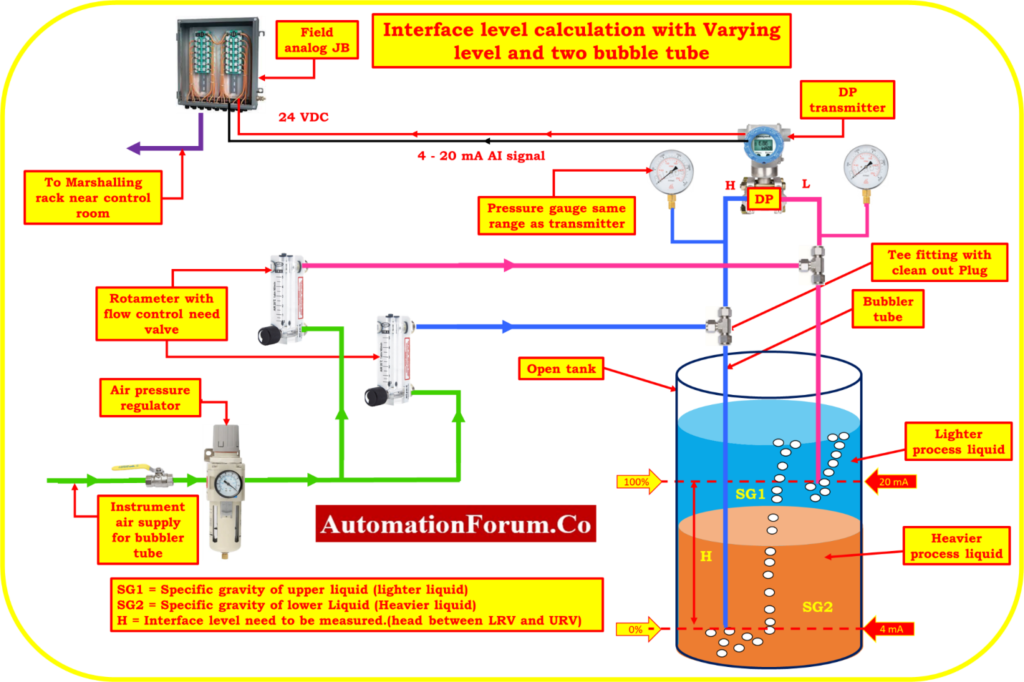# Bubbler method Interface level measurement Calculations with varying Tank Level and two Bubble TubesIn this section, examples of typical calculations for determining the liquid interface level in an open tank with with varying tank level and two bubble tubes transmitter installation are given.

It is important to keep in mind that the upper-range values (URV) and lower-range values (LRV) relate to the highest and minimum measurements that are intended, respectively. The Calibrated Span is equal to the URV minus the LRV. The output at LRV greater than 0% represents the value of the suppression.

## Abbreviations

The following acronyms are utilized in this directive.

LRV = Lower-range value (a measurement that’s output is 4 mA).

URV = Upper-range value (a measurement with a 20 mA output).

SG = A liquid’s specific gravity (relative density). Water has a specific gravity of 1.00 in both traditional and SI systems.

HW= Equivalent Headlevel pressure of Water

## Formulas for Converting Specific Gravity for All Liquids

Specific gravity = Density [lb/ft3 ] / 62.4

Specific gravity = Density [kg/m3] / 1.00

## Interface level measurement Calculations with varying Tank Level and two Bubble Tubes

SG1 = Specific gravity of upper liquid (lighter liquid)

SG2 = Specific gravity of lower Liquid (Heavier liquid)

H = Interface level need to be measured.(Head between LRV and URV)

Calibrated Range of the transmitter = LRV to URV

Where:

HW = Equivalent Head level pressure of Water

Span value of the transmitter = (H)*(SG2 – SG1)

Equivalent Head level pressure of Water (HW) at Lower range value

HW at LRV = (H*SG1) = Suppression

Equivalent Head level pressure of Water (HW) at Upper range value

HW at URV = (H*SG2)

## Example calculation – Interface level Calculations With Varying Tank Level and two Bubble Tubes

The DP transmitter is mounted in an open tank with two bubble tubes and also has the following specifications to determine interface level.

Process liquid Specific gravity varies between 1.0 and 1.7

Height of the interface level measured between LRV and URV is

H= 50 inches

Find the calibration range of the transmitter for measuring the interface level?

So, SG1 = 1.0

SG2= 1.7

## Span value calculation

Span = (H)*(SG2 – SG1)

Span = (50)*(1.7 – 1.0)

Span = (50)*(0.7)

Span value of the transmitter = 35 inches of H2O

## LRV calculation (4mA)

HW at LRV = (H*SG1)

HW at LRV = (50 *1.0)

HW at LRV= 50 inches of H2O = Suppression

## URV calculation (20mA)

HW at URV = (H*SG2)

Hw at URV = 50 * (1.7)

HW at URV = 85 inches of H2O

Calibration Range of the transmitter = 50 to 85 inches of H2O

Scroll to Top